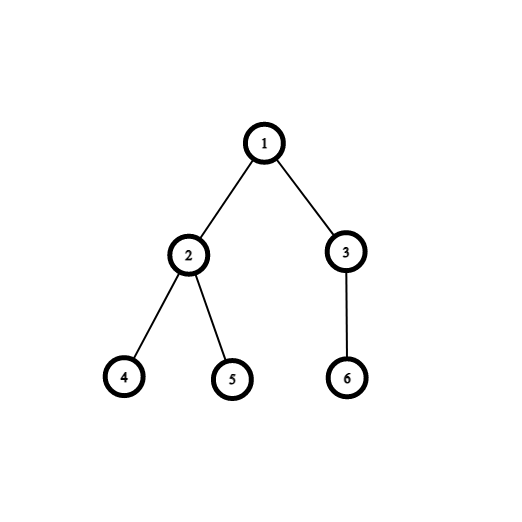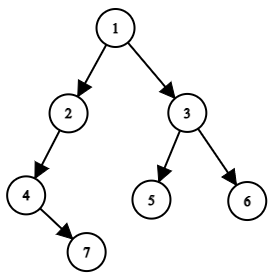# Nodes In Complete Binary Tree

Posted: 25 Mar, 2021
Difficulty: Moderate

## PROBLEM STATEMENT

#### A complete binary tree is a tree in which all the levels are completely filled except the last level. Nodes in the last level are as left as possible.

##### For Example:``````In the above complete binary tree, all the levels are filled except for the last. In the last level, all the nodes in the last level are as far left as possible.
``````
##### Input Format:
``````The first line contains an Integer 'T' which denotes the number of test cases or queries to be run. Then the test cases follow.

The first line of each test case contains elements of the tree in the level order form. The line consists of values of nodes separated by a single space. In case a node is null, we take -1 in its place.
````````````For example, the input for the tree depicted in the above image would be:

1
2 3
4 -1 5 6
-1 7 -1 -1 -1 -1
-1 -1
``````

#### Explanation:

``````Level 1 :
The root node of the tree is 1.

Level 2 :
Left child of 1 = 2
Right child of 1 = 3

Level 3 :
Left child of 2 = 4
Right child of 2 = null (-1)
Left child of 3 = 5
Right child of 3 = 6

Level 4 :
Left child of 4 = null (-1)
Right child of 4 = 7
Left child of 5 = null (-1)
Right child of 5 = null (-1)
Left child of 6 = null (-1)
Right child of 6 = null (-1)

Level 5 :
Left child of 7 = null (-1)
Right child of 7 = null (-1)
``````
##### Note:
``````The above format was just to provide clarity on how the input is formed for a given tree.

The sequence will be put together in a single line separated by a single space. Hence, for the above-depicted tree, the input will be given as:

1 2 3 4 -1 5 6 -1 7 -1 -1 -1 -1 -1 -1
``````
##### Output Format:
``````Return the number of nodes of the given complete
binary tree.
``````

#### Constraints:

``````1 <= T <= 10
0 <= Number of nodes in tree <= 10^5
1 <= Nodes Value <= 5*10^5

Time Limit: 1 second
``````Approach 1

Explanation:

The key idea is to traverse all the nodes of the tree and return the count of the number of nodes traversed.

Algorithm:

• First, check whether the root is null or not. If the root is null then return 0 (since the given tree is empty).
• Call recursion on the left subtree and right subtree. It will return the number of nodes in the left subtree and right subtree. Now return answer from left subtree + answer from right subtree + 1 (including the root node also).